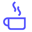a concise shot of dev knowledge
Become a Contributor
Concise shots of dev knowledge

RELATED TAGS

python
c++

# How to implement heap sortEdpresso Team

Heap sort is a sorting algorithm that takes the input data and sorts it in ascending or descending order. A Heap is a tree-like structure which is built using the input data.

The algorithm requires 3 steps to complete the sorting procedure:

1. Create a heap with the input data.

2. Sort the elements of the heap are sorted in ascending order.

3. Swap the root node with the last node and delete the last node from the heap.

Following slides will assist you in understanding this concept better:

1 of 17

## Code

The following codes implement the heap sort algorithm in C++ and Python.

#include <iostream>
using namespace std;

// To heapify a subtree rooted with node i which is
// an index in arr[]. n is size of heap
void heapify(int arr[], int n, int i)
{
int largest = i; // set largest as root
int l = 2 * i + 1; // left = 2*i + 1
int r = 2 * i + 2; // right = 2*i + 2

// If left child is larger than root
if (l < n && arr[l] > arr[largest])
largest = l;

// If right child is larger than largest so far
if (r < n && arr[r] > arr[largest])
largest = r;

// If largest is not root
if (largest != i) {
swap(arr[i], arr[largest]);

// Recursively heapify the affected sub-tree
heapify(arr, n, largest);
}
}

// main function to do heap sort
void heapSort(int arr[], int n)
{
// Build heap (rearrange array)
for (int i = n / 2 - 1; i >= 0; i--)
heapify(arr, n, i);

// One by one extract an element from heap
for (int i = n - 1; i >= 0; i--) {
// Move current root to end
swap(arr, arr[i]);

// call max heapify on the reduced heap
heapify(arr, i, 0);
}
}

// A function to print array
void printArray(int arr[], int n)
{
for (int i = 0; i < n; ++i)
cout << arr[i] << " ";
cout << "\n";
}

// Driver program
int main()
{
int arr[] = {4, 10, 3, 5, 1};
int n = sizeof(arr) / sizeof(arr);

heapSort(arr, n);

cout << "Sorted array is \n";
printArray(arr, n);
}


RELATED TAGS

python
c++
RELATED COURSES

View all Courses

Keep Exploring

Learn in-demand tech skills in half the time